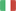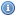EnglishItalianoVisitatore
Torna allaHomepage...

###Generali:

• Dipartimento: Scienze Matematiche, Fisiche E Naturali
• Tipologia: Corso Di Laurea Dm.270/04
• Corso di Laurea: Chimica
• Settore Ministeriale: MAT/05
• Codice di verbalizzazione: 8067298
• Metodi di insegnamento: Frontale
• Metodi di valutazione: Scritto E Orale
• Prerequisiti: Mathematical Analysis I
• Obiettivi: Students will learn basic notions on series, differential and integral calculus for real-valued functions of several real variables, and linear algebra. They will be able to apply them to calculate multidimensional integrals, to solve simple ordinary differential equations and to study systems of linear equations and power series. KNOWLEDGE AND UNDERSTANDING: Intuitive understanding of basic concepts of infinitesimal calculus and linear algebra, with some examples of mathematical formalization. APPLYING KNOWLEDGE AND UNDERSTANDING:Students will be able to compute partial derivatives, double, triple, curvilinear and surface integrals; to solve simple differential equations and linear systems; to study a numerical or power series; they will be able to understand the mathematical formalization of a problem. MAKING JUDGEMENTS: Students will be able to figure out the solution method for simple mathematical problems. COMMUNICATION SKILLS: Students will learn how to explain the mathematical procedure chosen to solve a problem. LEARNING SKILLS: Students will learn how to distinguish the essential features of a mathematical problem and how to study from a textbook effectively.
• Ricevimento: Mar 13-15

###Didattica:

• A.A.: 2020/2021
• Canale: UNICO
• Crediti (CFU): 9
• Obbligo di Frequenza: No